# Physics Homework Help With Rotational Kinetic Energy

1. Home
2. Physics
3. Rotational Motion
4. Torque
5. Rotational Kinetic Energy

## Rotational Kinetic Energy

Consider a rigid body rotating about a fixed axis with angular velocity w. The body can be supposed to be made up of a large number of particles m1, m2, ..., mn at distances r1, r2, ..., rn respectively, from the axis of rotation. Each particle has a kinetic energy. The kinetic energy of the body will be the sum of the kinetic energies of all the particles. If u1, u 2, ..., un be the linear speeds of the particles, respectively, then the kinetic energy of the body can be written as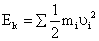But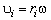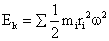=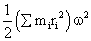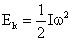where I is the moment of inertia of the body. From this expression it is clear that moment of inertia is the rotational analogue of mass.

### Homework Help For Rotational Kinetic Energy

assignmenthelp.net provides best Online Assignment Help service in Physics for all standards. Our Tutor provide their high quality and optimized Tutorial help to fulfill all kind of need of Students.